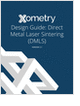Formula HomePlate TheoryClassical PlateKinematicsConstitutiveResultantsEquilibriumSign ConventionPlate CalculatorsCalculator ListMaterial DataElastic ModuliResourcesBibliography
Essentials of Manufacturing

Information, coverage of important developments and expert commentary in manufacturing.

Metal 3D Printing Design Guide

Direct Metal Laser Sintering (DMLS) 3D printing for parts with reduced cost and little waste.

3D Scanners

A white paper to assist in the evaluation of 3D scanning hardware solutions.

Learn the best principles to negotiate the salary you deserve!

more free publicationsChoose a Boundary Condition and Calculate!Simply Supported(SSSS) Free-SSS(FSSS) Clamped (Fixed)(CCCC) Free-CCC(FCCC)Simply Supported(cpS) Free-Simply Supported(cpFS) Clamped (Fixed)(cpC) Free-Clamped(apFC)Classical Plate EquationThe small transverse (out-of-plane) displacement w of a thin plate is governed by the Classical Plate Equation,where p is the distributed load (force per unit area) acting in the same direction as z (and w), and D is the bending/flexual rigidity of the plate defined as follows,in which E is the Young's modulus,is the Poisson's ratio of the plate material, and t is the thickness of the plate. Furthermore, the differential operatoris called the Laplacian differential operator,If the bending rigidity D is constant throughout the plate, the plate equation can be simplified to,whereis called the biharmonic differential operator. This small deflection theory assumes that w is small in comparison to the thickness of the plate t, and the strains and the midplane slopes are much smaller than 1. A plate is called thin when its thickness t is at least one order of magnitude smaller than the span or diameter of the plate.
 Origin of the Plate EquationThe classical plate equation arises from a combination of four distinct subsets of plate theory: the kinematic, constitutive, force resultant, and equilibrium equations. The outcome of each of these segments is summarized here:
 Kinematics:where w0 is the displacement of the middle plane in z direction. Constitutive:Resultants:Equilibrium:To relate the plate's out-of-plane displacement w0 to its pressure loading pz, we combine the results of the four plate subcategories in this order:
 Kinematics -> Constitutive -> Resultants -> Equilibrium = PlateEquation
 The plate is assumed to be constructed by isotropic material and subjected to transverse loading. Also, the Cartesian coordinate system is used. We'll demonstrate this hierarchy by working backwards. We first combine the 3 equilibrium equations to eliminate Qxz and Qyz,Next, replace the moment resultants with its definition in terms of the direct stress,Note that uniform thickness is assumed. Use the constitutive relation to eliminate stress in favor of the strain,and then use kinematics to replace strain in favor of the normal displacement w0,The equation of equilibrium can then be expressed in terms of the normal displacement w0which yieldsNote that homogeneous material across the plate (x and y directions) is assumed. As a final step, assuming homogeneous material along the thickness of the plate, the bending stiffness of the plate can be written asWe then arrive at the Classical Plate equation,or a slimmer formwhere w0 is replaced by w and pz replaced by p to be consistent with the notations in most published literatures.Essentials of Manufacturing

Information, coverage of important developments and expert commentary in manufacturing.Metal 3D Printing Design Guide

Direct Metal Laser Sintering (DMLS) 3D printing for parts with reduced cost and little waste.3D Scanners

A white paper to assist in the evaluation of 3D scanning hardware solutions.